# Selina Concise Mathematics Class 9 ICSE Solutions Rectilinear Figures [Quadrilaterals: Parallelogram, Rectangle, Rhombus, Square and Trapezium]

## Selina Concise Mathematics Class 9 ICSE Solutions Rectilinear Figures [Quadrilaterals: Parallelogram, Rectangle, Rhombus, Square and Trapezium]

APlusTopper.com provides step by step solutions for Selina Concise Mathematics Class 9 ICSE Solutions Chapter 14 Rectilinear Figures [Quadrilaterals: Parallelogram, Rectangle, Rhombus, Square and Trapezium]. You can download the Selina Concise Mathematics ICSE Solutions for Class 9 with Free PDF download option. Selina Publishers Concise Mathematics for Class 9 ICSE Solutions all questions are solved and explained by expert mathematic teachers as per ICSE board guidelines.

Selina ICSE Solutions for Class 9 Maths Chapter 14 Rectilinear Figures [Quadrilaterals: Parallelogram, Rectangle, Rhombus, Square and Trapezium]

Exercise 14(A)

Solution 1:Solution 2:Solution 3: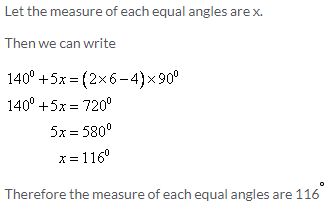Solution 4: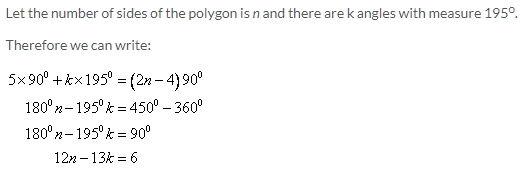In this linear equation n and k must be integer. Therefore to satisfy this equation the minimum value of k must be 6 to get n as integer.
Hence the number of sides are: 5 + 6 = 11.

Solution 5: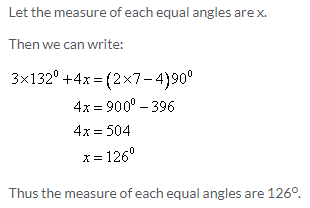Solution 6: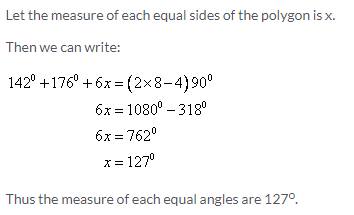Solution 7:Solution 8: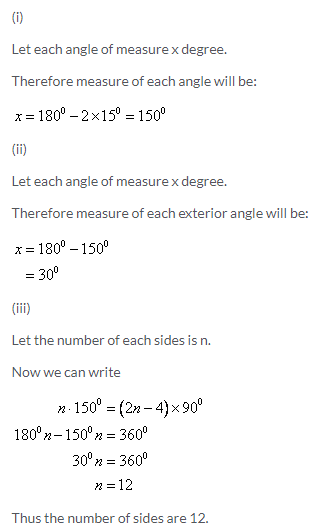Solution 9:Solution 10:Solution 11:Exercise 14(B)

Solution 1:
(i)True.
This is true, because we know that a rectangle is a parallelogram. So, all the properties of a parallelogram are true for a rectangle. Since the diagonals of a parallelogram bisect each other, the same holds true for a rectangle.
(ii)False
This is not true for any random quadrilateral. Observe the quadrilateral shown below.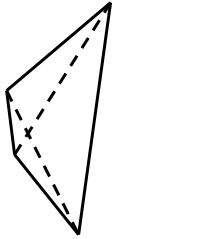Clearly the diagonals of the given quadrilateral do not bisect each other. However, if the quadrilateral was a special quadrilateral like a parallelogram, this would hold true.
(iii)False
Consider a rectangle as shown below.It is a parallelogram. However, the diagonals of a rectangle do not intersect at right angles, even though they bisect each other.
(iv)True
Since a rhombus is a parallelogram, and we know that the diagonals of a parallelogram bisect each other, hence the diagonals of a rhombus too, bisect other.
(v)False
This need not be true, since if the angles of the quadrilateral are not right angles, the quadrilateral would be a rhombus rather than a square.
(vi)True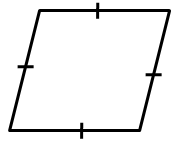A parallelogram is a quadrilateral with opposite sides parallel and equal.
Since opposite sides of a rhombus are parallel, and all the sides of the rhombus are equal, a rhombus is a parallelogram.
(vii)False
This is false, since a parallelogram in general does not have all its sides equal. Only opposite sides of a parallelogram are equal. However, a rhombus has all its sides equal. So, every parallelogram cannot be a rhombus, except those parallelograms that have all equal sides.
(viii)False
This is a property of a rhombus. The diagonals of a rhombus need not be equal.
(ix)True
A parallelogram is a quadrilateral with opposite sides parallel and equal.
A rhombus is a quadrilateral with opposite sides parallel, and all sides equal.
If in a parallelogram the adjacent sides are equal, it means all the sides of the parallelogram are equal, thus forming a rhombus.
(x)False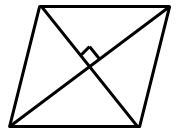Observe the above figure. The diagonals of the quadrilateral shown above bisect each other at right angles, however the quadrilateral need not be a square, since the angles of the quadrilateral are clearly not right angles.

Solution 2: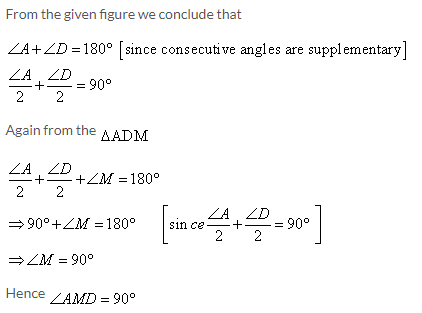Solution 3: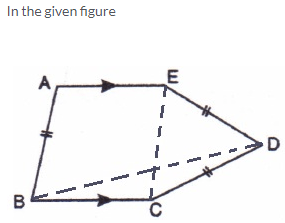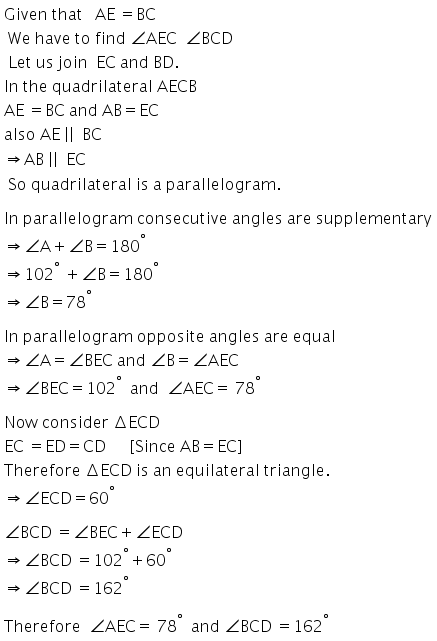Solution 4:Solution 5: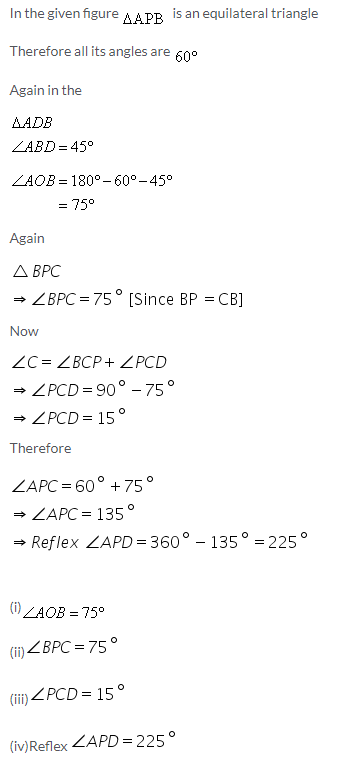Solution 6: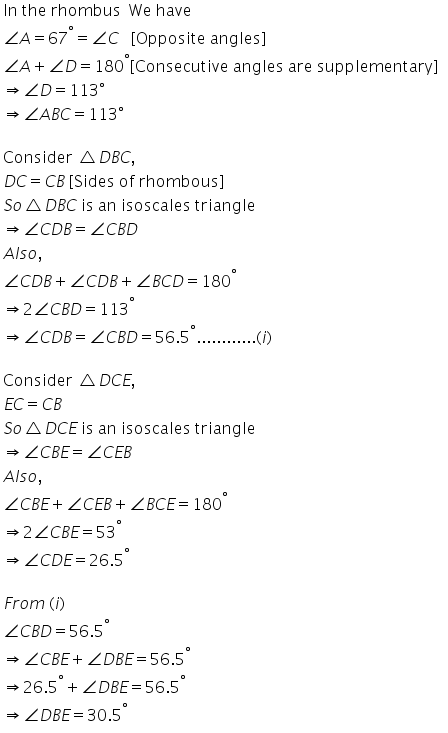Solution 7:Solution 8: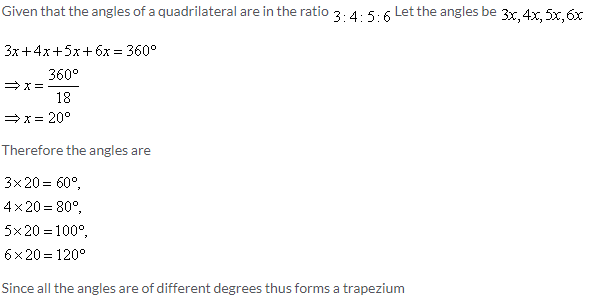Solution 9:Solution 10:
We know that AQCP is a quadrilateral. So sum of all angles must be 360.
∴ x + y + 90 + 90 = 360
x + y = 180
Given x:y = 2:1
So substitute x = 2y
3y = 180
y = 60
x = 120
We know that angle C = angle A = x = 120
Angle D = Angle B = 180 – x = 180 – 120 = 60
Hence, angles of parallelogram are 120, 60, 120 and 60.

Exercise 14(C)

Solution 1: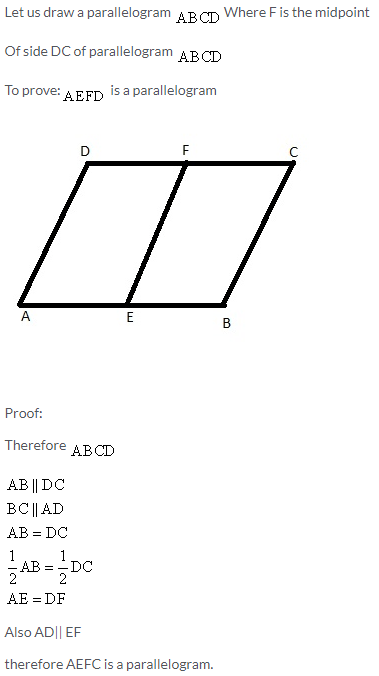Solution 2: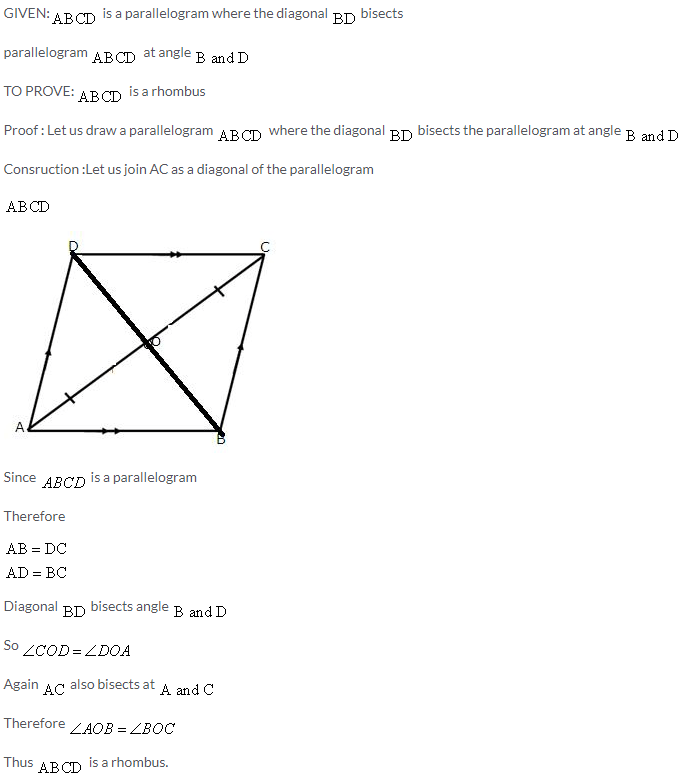Solution 3:Solution 4: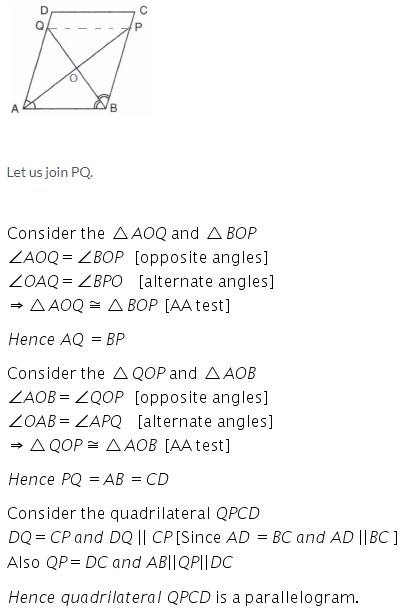Solution 5:Solution 6: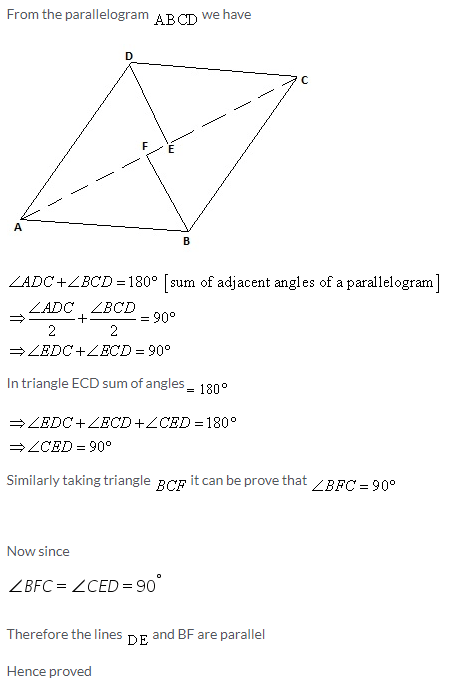Solution 7: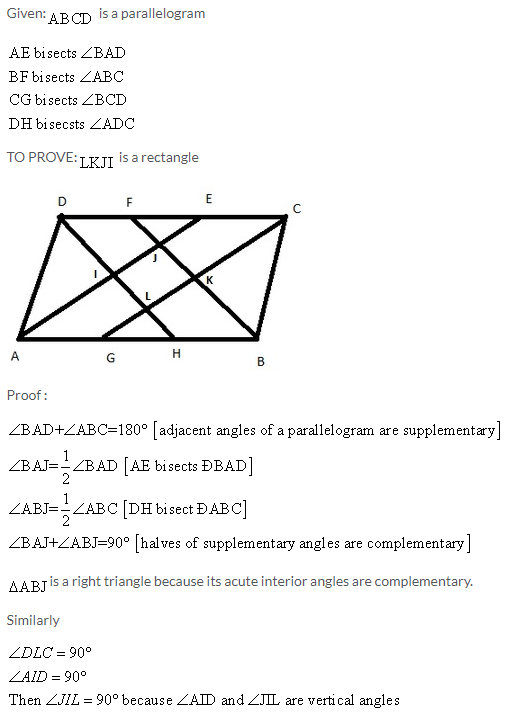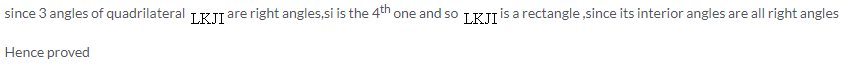Solution 8: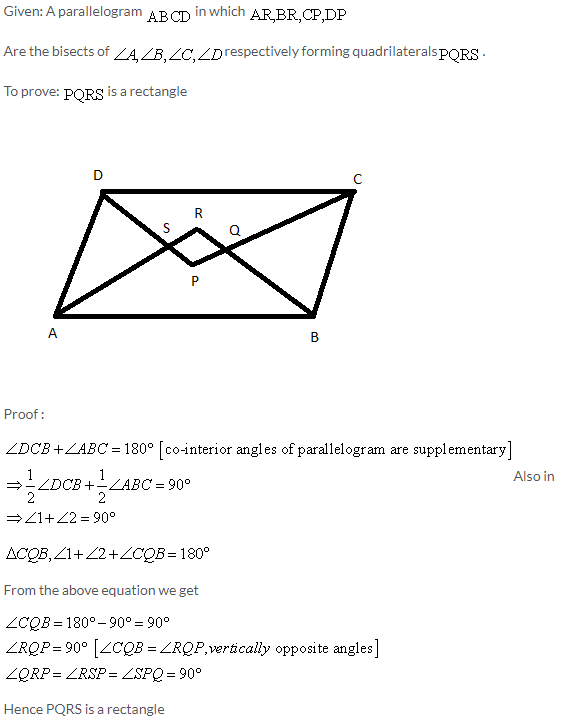Solution 9: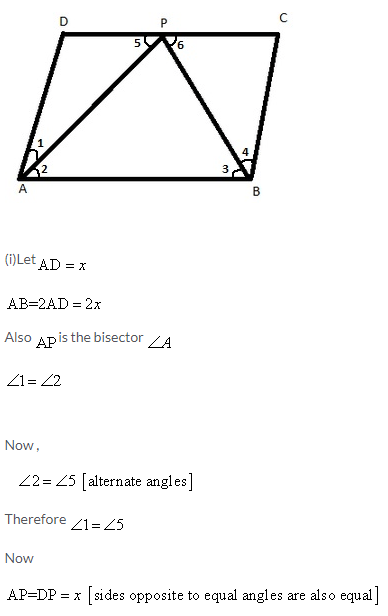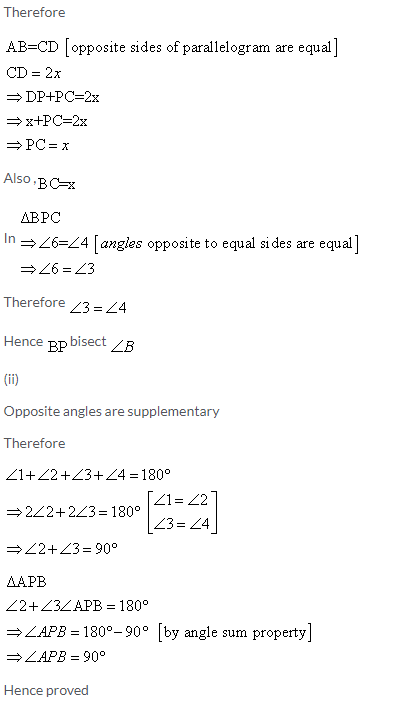Solution 10: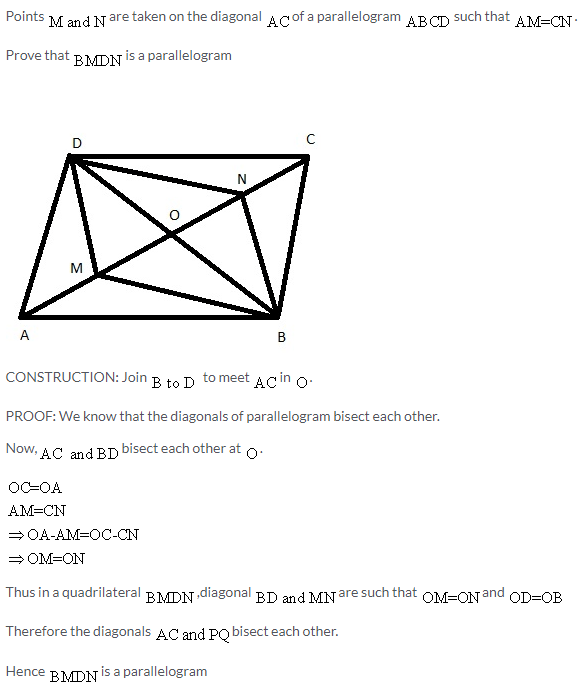Solution 11: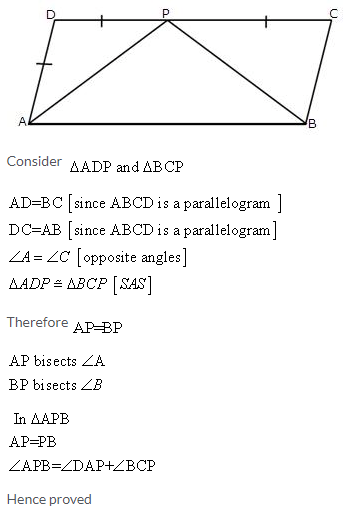Solution 12:Solution 13:Solution 14: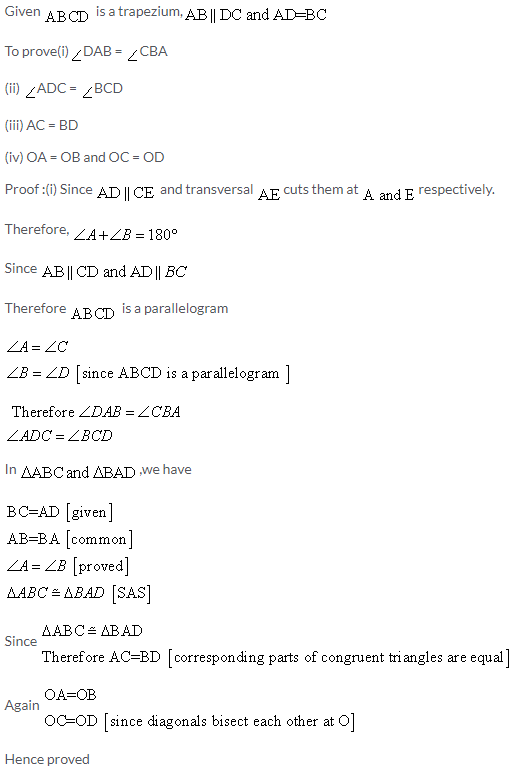Solution 15: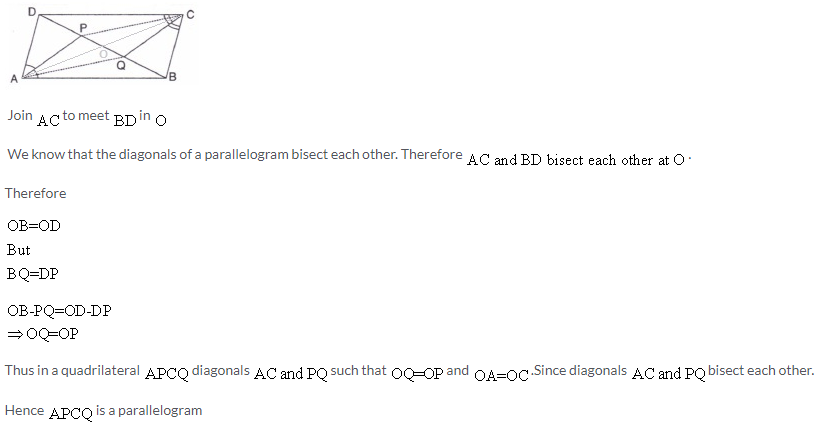Solution 16: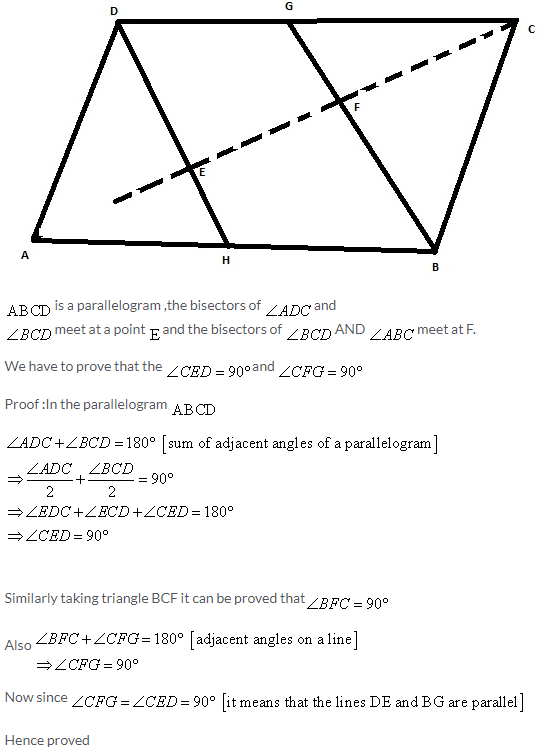Solution 17: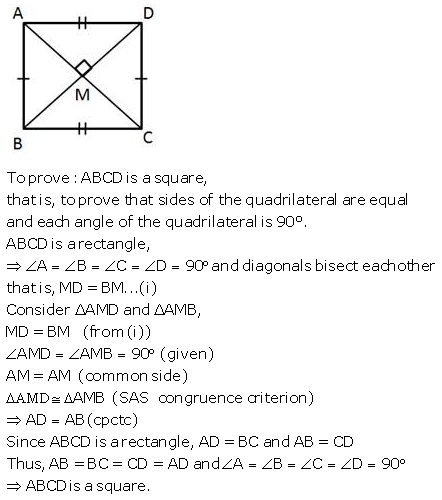Solution 18:Solution 19: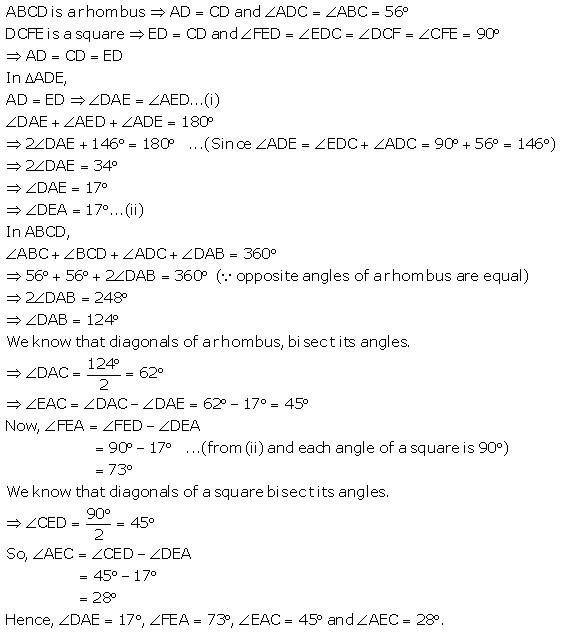More Resources for Selina Concise Class 9 ICSE Solutions